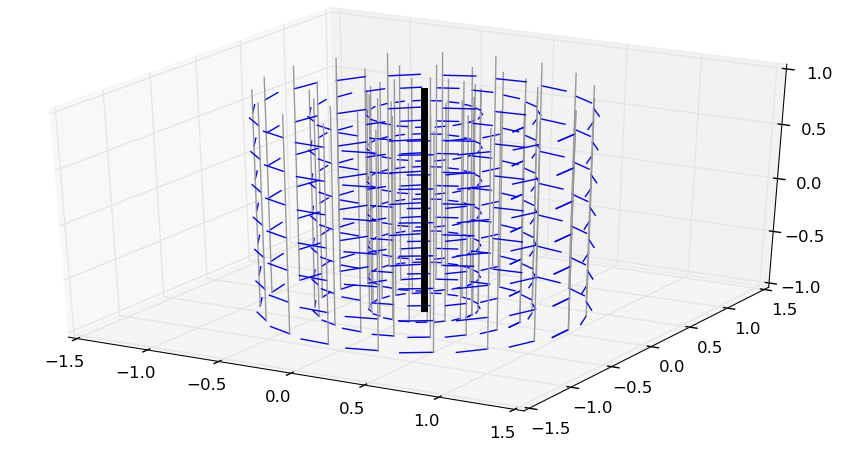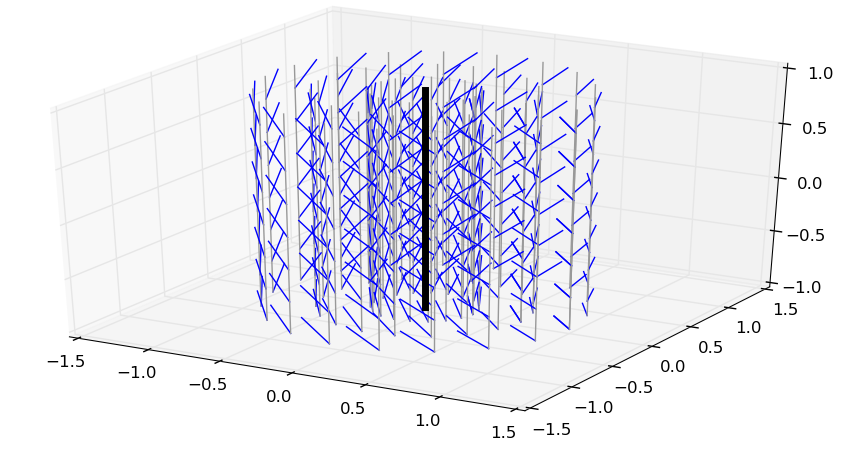# Screw axes

Screws, the mathematical objects used to describe rigid body motions and forces, are intrinsically linked with axes, be it axes of motion (such as the rotation axis of a pure rotation) or force (such as the line of action of a force).

## Central and noncentral axes

The central axis of a screw $$\hat\bfs$$ is the axis directed by the resultant $$\bfr$$ where the moment $$\bfm_P$$ is parallel to $$\bfr$$ (not necessarily zero). A noncentral axis of the screw is any other axis in space directed by the resultant $$\bfr$$. From Varignon’s formula, any two points $$P$$ and $$Q$$ belonging to a noncentral axis have the same moment $$\bfm_P = \bfm_Q$$. This way, the moment field can be seen as a vector field over noncentral axes (5D space) rather than over points (6D space).

Another interesting value for a screw is its automoment, which is the scalar product $$(\bfr \cdot \bfm_P)$$ between its resultant and its moment. Again, from Varignon’s formula, it does not depend on the point $$P \in \mathsf{E}^3$$ selected to compute it. In particular, on the central axis $$\Delta_c$$, the moment is given by:

\begin{equation*} \bfm_{\Delta_c} = (\bfr \cdot \bfm_{\Delta_c})\,\frac{\bfr}{\| \bfr \|^2} \ = \ \rho(\hat\bfs)\,\bfr \end{equation*}

where $$\rho(\hat\bfs) = (\bfr \cdot \bfm_P) / (\bfr \cdot \bfr)$$ is the pitch of the screw. A screw is fully defined by:

• its pitch $$\rho(\hat\bfs)$$,
• its central axis $$\Delta_c$$, and
• its magnitude $$\| \bfr \|$$.

Going back to Chasles’ theorem on twists:

• the central axis $$\Delta_c$$ is the axis of the motion,
• the magnitude $$\| \bfomega \|$$ gives the amount of the rotation around $$\Delta_c$$, and
• the pitch $$\rho(\hat\bft) = (\bfomega \cdot \bfv_P) / (\bfomega \cdot \bfomega)$$ gives the amount of translation along $$\Delta_c$$.

## Illustration

Here is a small Python function with mplot3d to plot the moment field of a screw, assuming $$\Delta_c$$ goes through the origin:

from matplotlib.pyplot import figure, show, ion
from mpl_toolkits.mplot3d import Axes3D
from numpy import linspace, cos, sin, arange, pi, array, cross

def plot_screw(res, pitch, scale=0.2):
"""
Plot screw as a vector field.

Args:
res (float): size of each arrow in our plot.
pitch (float): pitch of the screw.
scale (float): scaling factor for each arrow.
""""
fig = figure()
ax = fig.gca(projection='3d')
for r in linspace(0., 1., 4):
for theta in linspace(0, 2 * pi, 20):
x, y = r * cos(theta), r * sin(theta)
ax.plot([x - res, x + res], [y - res, y + res],
[-1, 1], color='#999999')
for z in arange(-1., 1., 0.3):
u, v, w = scale * (cross([x, y, z], res) + pitch * res)
ax.plot([x, x + u], [y, y + v], [z, z + w], color='b')
ax.plot([-res, +res], [-res, +res], [-1, 1], color='k', lw=5)
show()


Here is the example of a screw with zero pitch, such as a pure rotation. The bold black line shows the central axis, while the thin gray lines are for noncentral ones. The moment field (linear velocities) is in blue.Now, the same with a pitch $$\rho(\hat\bft) = 1$$ m/rad, meaning each radian of rotation brings a one meter translation:We see where the word “screw” comes from: following the moment field of the screw above is like following the thread of an actual screw.

## To go further

An excellent read is Sardain and Bessonnet’s survey paper Forces Acting on a Biped Robot. Center of Pressure—Zero Moment Point. It uses of the axial representation of screws to derive the center of pressure and zero-tilting moment point of the contact wrench, which are commonly used in balance control of legged robots.

## Discussion

You can use Markdown with $\LaTeX$ formulas in your comment.